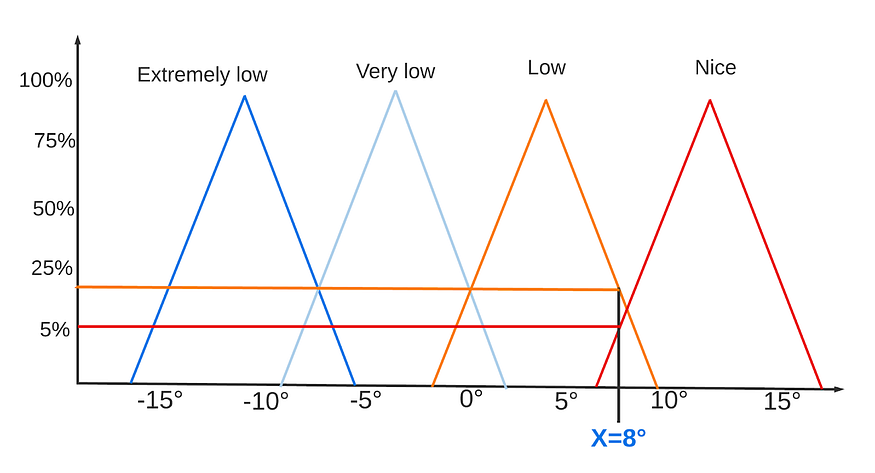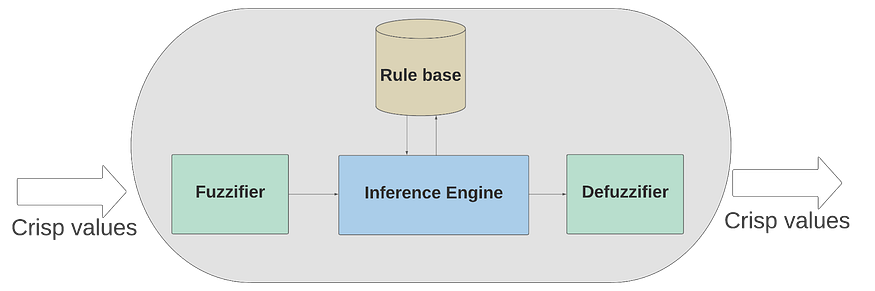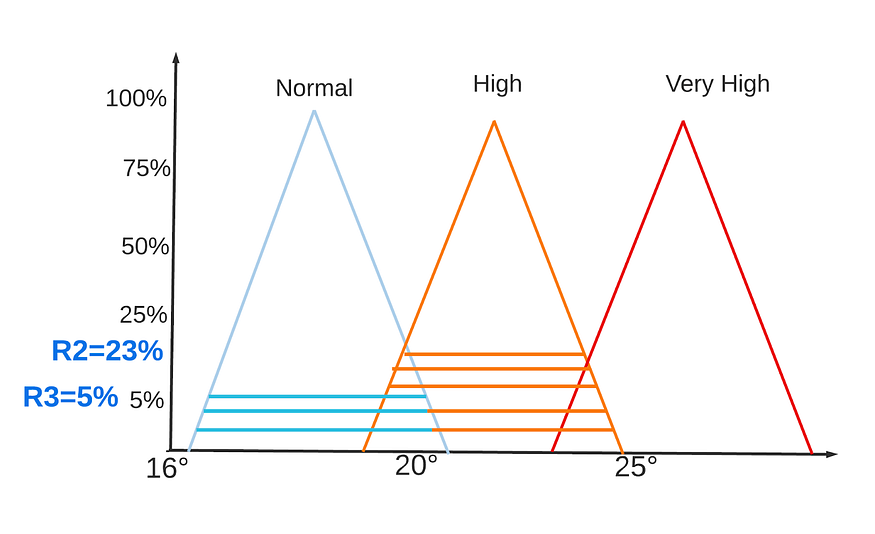This is the second part of the fuzzy logic and machine learning interpretability article series.

In the previous part (here) we discussed Machine learning interpretability; its definition(s), taxonomy of its methods and its evaluation methodologies.

In this second part, we will introduce fuzzy logic and its main components: fuzzy sets, membership functions, linguistic variables, and fuzzy inference systems. These concepts are crucial to understand the use of fuzzy logic in machine learning interpretability.

## Limitations of crisp sets, and the need for fuzzy sets

Classical (or crisp) set theory is one of the most fundamental concepts of mathematics and science. It is an essential tool in various domains, and especially in computer science (e.g., databases, data structures).

Classical set theory relies on the notion of dichotomy: an element x either belongs to a set S or does not. In fact, one of the defining features of a crisp set is that it classifies the elements of a given universe of discourse to members and nonmembers. Crisp sets have clear and unambiguous boundaries.

The belongingness of an element to a set is expressed via a characteristic function.

A characteristic function S(x) of a set S defined on the universe of discourse X has the following form:

(1) S(x)=1 , if x S and 0 , otherwise

Despite its widespread use, classical set theory still has several drawbacks.

Among them is its incapacity to model imprecise and vague concepts. For instance, let’s say we want to define the set tall people using crisp sets, and we decided to use the following characteristic function:

(2) tallpeople =1 if height>175, 0 otherwise

Meaning that a person is a member of the set of tall people, if their height is greater than 1.75 meters. This definition of the concept of tall leads to unreasonable conclusions. For example, using the characteristic function (2) we deduce that a person whose height is 174.9 cm is not tall, while a person whose height is 175.1 cm is. This sharp transition between inclusion and exclusion sets is one of crisp sets theory’s major flaws, And it is what has led to development and the use of fuzzy sets theory.

## Fuzzy logic and fuzzy sets

“As complexity rises, precise statements lose meaning and meaningful statements lose precision”, Lotfi A. Zadeh

Fuzzy logic attempts to resemble the human decision-making process. It deals with vague and imprecise information. Fuzzy logic-based systems have shown great potential in dealing with problems where information is vague and imprecise. They are being used in numerous applications ranging from voice recognition to control.

Fuzzy set theory (introduced in 1965 by Lotfi Zadeh) is an extension of classical set theory. Fuzzy set theory departs from the dichotomy principle and allows for memberships to a set to be a matter of degree. It has gained so much ground because it models better the physical world where most of the knowledge is fuzzy and vague. For example, notions like good, bad, tall, and young are all fuzzy. That is, there is neither a qualitative value, nor a range with clear boundaries that defines them.

## Membership functions

Formally, a fuzzy set F is characterized by a membership function mapping the elements of a universe of discourse to the interval [0, 1].

F :X0,1

Membership functions map elements of the universe of discourse X to an interval [0,1] where 0 means exclusion, 1 signifies complete membershipand the intermediate membership values show that an element can be a member of two classes at the same time with different degrees. This allows gradual transitions from one class to the other.

The belonging to multiple classes at the same time, and the gradual transition from one class to another, are illustrated in the following example.

Figure 1 shows membership functions of the concept Temperature. In this example, a temperature in celsius can be a member of the following fuzzy sets : Extremely Low, Very low, Low, and Nice temperatures. The X-axis and Y-axis refer respectively to the temperature values in celsius and belongingness percentages. For example, a temperature of 8° is 5% nice and 23% low.

This process of mapping crisp values (such as 8°) to fuzzy values is called Fuzzification. And it is the first step in fuzzy inference systems, more on that later.Figure 1. Membership functions of the fuzzy sets: extremely low, very low, low, nice temperatures

## Linguistic variables

Another fundamental concept of fuzzy logic is linguistic variables. Thanks to linguistic variables (or fuzzy variables), machines can understand the human language, and do inference using our vague and imprecise statements.

A linguistic variable can be defined as a variable whose values are not numbers, but rather, words. Formally, a linguistic variable is defined by the following quintuple:

• X: the name of the variables
• T(X): the set of terms of X
• U: the universe of discourse
• G: the syntactic rule that generates the name of the terms
• µ: the semantic rule that associates each term of X with its meaning

For example, suppose that X is a linguistic variable called Temperature, its set of terms T(X) can be {low, medium, high}, the universe of discourse U can be the interval [-20:55], and the semantic rules are the membership functions of each term.

## Fuzzy inference system

Fuzzy sets and linguistic variables allow us to model human knowledge better. However, can machines do inference using the expert knowledge we embedded in them using fuzzy logic?

Fuzzy inference systems do just that.

Given an input and output, a fuzzy inference system formulates a mapping using a set of fuzzy rules (fuzzy rules have the following form If A Then B, where A and B are fuzzy sets).

This mapping allows future decisions to be made and patterns to be discerned in a transparent manner. Fuzzy inference systems are composed of the following components:

• Fuzzifier: converts the crisp input values to fuzzy ones using the membership functions.
• Rule base: stores the fuzzy rules
• Inference engine: performs the fuzzy inference process
• Defuzzifier: transfers the fuzzy inference results into a crisp output.Figure 2. Main components of a fuzzy inference system.

There are mainly two groups of fuzzy inference systems: Mamdani, and Sugeno (TSK). The difference between the two lies in the consequent part of their fuzzy rules. The consequent part of the Mamdani fuzzy inference system is a fuzzy set. Meanwhile, the consequence of the TSK fuzzy inference system is a mathematical function. This allows the latter to have more predictive power, and the former to have more interpretability.

## Example of a fuzzy inference system

To better understand what a fuzzy inference system is, and what it does, let’s discuss the following example of a Mamdani based fuzzy inference system.

We will try to build a fuzzy inference system that takes as input a temperature value, and adjusts the temperature of the Air Conditioner (AC).

• Rule base and membership functions:

To build a fuzzy inference system, a rule base (i.e., a set of fuzzy rules) and membership functions (defining the fuzzy sets) need to be provided. They are usually provided by domain experts.

For the sake of simplicity, let’s use the membership functions in Figure 1. And let’s suppose that our rule base contains the following three rules:

Rule 1: if Temperature is Very Low or Extremely Low Then AC_Command is Very High

Rule 2: if Temperature is Low Then AC_Command is High

Rule 3: if Temperature is Nice Then AC_Command is Normal

• Fuzzification

The first operation that a fuzzy inference system conducts is fuzzification, i.e., it uses the membership functions to convert the crisp input values to fuzzy values.

If our system receives as input the value x=8°, using the membership functions we defined, this value means that the temperature is 5% nice and 23% low. Formally, this can be written as:

U(x=Low)=0.23

U(x=Nice)=0.05

U(x=Very Low)=0

U(x=Extremely Low)=0

• Rules evaluation:

Having fuzzified the input, we can now evaluate the rules in our rule base.

Rule 1: if Temperature is Very Low (=0) or Extremely Low (=0) Then AC_Command is Very High (=0)

Rule 2: if Temperature is Low (=0.23) Then AC_Command is High (=0.23)

Rule 3: if Temperature is Nice (=0.05) Then AC_Command is Normal (=0.05)

• Aggregation and defuzzification:

Aggregation is the process of unifying the outputs of all rules. The values found in the rule evaluation process (0.05 and 0.23) are projected onto the membership functions of AC_Command (Figure 3). The resulting membership functions of all rule consequent are combined.

Finally, defuzzification is carried out. One popular way to conduct defuzzification is to take the output distribution (blue and orange area in Figure 3) and compute its center of mass. This crisp number would be in our example the appropriate degree of the AC.Figure 3. membership functions of the fuzzy sets Normal, High, Very High AC command

## Conclusion

This article covered the basic notions underlying fuzzy logic. We saw how the need for fuzzy sets emerged, and we briefly discussed what fuzzy sets, membership functions, and linguistic variables are. The most important one of all the concepts we saw in this blog (to understand how fuzzy logic is used in machine learning interpretability) is probably fuzzy inference systems.

In the next article (the final), everything we discussed so far will be brought together (ML interpretability and fuzzy logic) to present and discuss what Neuro-Fuzzy architectures are, and how they can be used to tackle the interpretability-performance tradeoff.

## References

Department of Computer Engineering, Sharif University of Technology — Fuzzy logic course

Biosignal Processing and Classification Using Computational Learning and Intelligence- Chapter 8 — Fuzzy logic and fuzzy systems

1. A. Kalogirou, “Solar Energy Engineering: Processes and Systems: Second Edition,” Solar Energy Engineering: Processes and Systems: Second Edition, pp. 1–819, 2014, doi: 10.1016/C2011–0–07038–2.
2. Zhang et al., “Neuro-Fuzzy and Soft Computing — A Computational Approach to Learning and Artificial Intelligence,” International Review of Automatic Control (IREACO), vol. 13, no. 4, pp. 191–199, Jul. 2020, doi: 10.15866/IREACO.V13I4.19179.
More Tags
Share